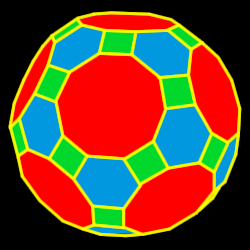Truncated Icosidodecahedron

Properties of the truncated icosidodecahedron: Number of faces, edges and dihedral angle measure

The truncated icosidodecahedron is created by truncating (cutting off) the icosidodecahedron one third of the way into each side62 total faces:
30 squares, 20 regular hexagons and 12 regular decagons

120 vertices:
1 square, 1 hexagon and 1 decagon

180 edges

Dihedral angles
148 degrees, 17 minutes for the dec-sqr angle,
142 degrees, 37 minutes for the dec-hex angle and 159 degrees, 6 minutes for the hex-sqr angle